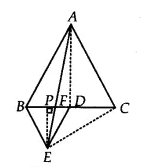Q

# In Fig.9.33, ABC and BDE are two equilateral triangles such that D is the mid-point of BC. If AE intersects BC at F, show that (i) ar (BDE) = 1/4 ar (ABC)

Q: 5    In Fig. , ABC and BDE are two equilateral triangles such that D is the mid-point of BC. If AE intersects BC at F, show that

(i)

[Hint: Join EC and AD. Show that and    , etc.]ViewsLet join the CE and AD and draw . It is given that ABC and  BDE is an equilateral triangle.
So,   AB =BC = CA =   and D i sthe midpoint of BC
therefore,
(i) Area of  ABC =  and
Area of BDE =

Hence,

Exams
Articles
Questions